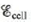# Calculate the pH of the cathode compartment for the following reaction given = 3.01 V when [Cr 3+ ] = 0.15 M , [Al 3+ ] = 0.30 M, and [Cr 2 O 7 2− ] = 0.55 M . 2Al ( s ) + Cr 2 O 7 2 − ( a q ) + 14H + ( a q ) → 2A1 3 + ( a q ) + 2Cr 3 + ( a q ) + 7H 2 O ( l )### Chemistry: An Atoms First Approach

2nd Edition
Steven S. Zumdahl + 1 other
Publisher: Cengage Learning
ISBN: 9781305079243

#### Solutions

Chapter
Section### Chemistry: An Atoms First Approach

2nd Edition
Steven S. Zumdahl + 1 other
Publisher: Cengage Learning
ISBN: 9781305079243
Chapter 17, Problem 72E
Textbook Problem
1 views

## Calculate the pH of the cathode compartment for the following reaction given= 3.01 V when [Cr3+] = 0.15 M, [Al3+] = 0.30 M, and [Cr2O72−] = 0.55 M. 2Al ( s )   +  Cr 2 O 7 2 − ( a q ) +  14H + ( a q ) →  2A1 3 + ( a q ) +  2Cr 3 + ( a q ) +  7H 2 O ( l )

Interpretation Introduction

Interpretation:

The chemical reaction for a galvanic cell is given. The pH of the cathode compartment for the given reaction is to be calculated.

Concept introduction:

The H+ ion concentration is measured by the value of pH . Higher pH corresponds to a basic solution while the lower value corresponds to acidic solution. The value of pH is also demonstrated using the Nernst equation.

The Nernst formula is defined as follows,

E=E°(RTnF)ln(Q)

To determine: The value of E at 25°C for this battery at the given concentrations of different ions.

### Explanation of Solution

Given

The value of concentration of Cr3+ is 0.15M .

The value of concentration of Al3+ is 0.30M .

The value of concentration of Cr2O72 is 0.55M .

The value of Ecell is 3.01V .

The reaction taking place at cathode is,

Cr2O72(aq)+14H+(aq)+6e2Cr3+(aq)+7H2O(l)E°red=1.33V

The reaction taking place at anode is,

AlAl3++3eE°ox=1.66V

Multiply the oxidation half with a coefficient of 2 and then add both the oxidation and reduction half reaction,

Cr2O72(aq)+14H+(aq)+6e2Cr3+(aq)+7H2O(l)2Al2Al3++6e

The final equation is,

Cr2O72(aq)+2Al(s)+14H+(aq)2Cr3+(aq)+2Al3+(aq)+7H2O(l)

The value of E°cell is calculated as,

E°cell=E°ox+E°red=1.66V+1.33V=2.99V_

The reaction involves the transfer of 6 moles of electrons and the value of Ecell=3

### Still sussing out bartleby?

Check out a sample textbook solution.

See a sample solution

#### The Solution to Your Study Problems

Bartleby provides explanations to thousands of textbook problems written by our experts, many with advanced degrees!

Get Started

Find more solutions based on key concepts
People who take lip physical activity for weight loss can increase weight loss by using sports drinks do not ne...

Nutrition: Concepts and Controversies - Standalone book (MindTap Course List)

Match the term listed in Column A with its definition from Column B

Nutrition Through the Life Cycle (MindTap Course List)

Express the following quantities using the prefixes given in Table 1.4. (a) 3 10-4 (b) 5 10-5 s (c) 72 102 g

Physics for Scientists and Engineers, Technology Update (No access codes included)